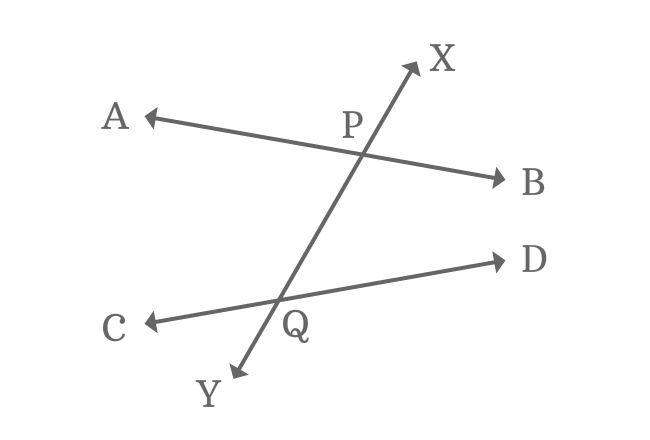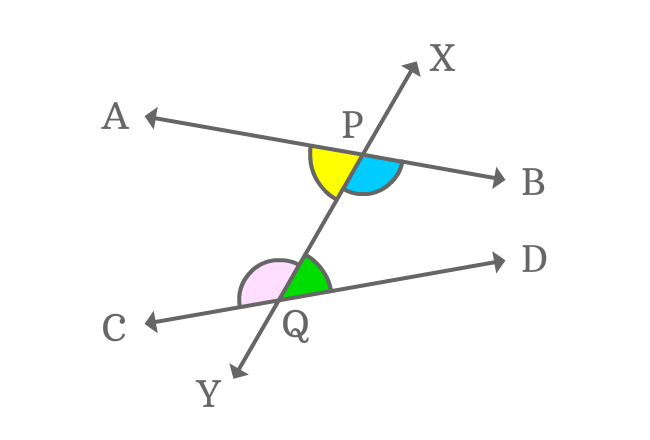# Alternate Interior Angles formed by Nonparallel Lines and their transversalThe interior angles which appear opposite sides of transversal of nonparallel lines are called the alternate interior angles formed by nonparallel lines and their transversal line.

When two nonparallel lines are intersected by their transversal, four interior angles are formed geometrically but every two of them are appeared opposite sides at the intersection of the non-parallel lines by their transversal line. Hence, two pairs of alternate interior angles are formed by the intersection.

## Alternate Interior AnglesWhen two nonparallel lines ($\overleftrightarrow{AB}$ and $\overleftrightarrow{CD}$) are intersected by their transversal line $\overleftrightarrow{XY}$, four interior angles $\angle YPA$, $\angle YPB$, $\angle XQC$ and $\angle XQD$ are formed geometrically.

The following two pairs of interior angles are alternate interior angles.

$1. \,\,\,\,\,\,$ $\angle YPA$ and $\angle XQD$

$2. \,\,\,\,\,\,$ $\angle YPB$ and $\angle XQC$

### PropertyEach pair of alternate interior angles are not equal. It is mainly due to the involvement of nonparallel lines in the intersection with their transversal line.

$1. \,\,\,\,\,\,$ $\angle YPA \,\ne\, \angle XQD$

$2. \,\,\,\,\,\,$ $\angle YPB \,\ne\, \angle XQC$

Latest Math Topics
Jun 26, 2023
Jun 23, 2023

###### Math Questions

The math problems with solutions to learn how to solve a problem.

Learn solutions

Practice now

###### Math Videos

The math videos tutorials with visual graphics to learn every concept.

Watch now

###### Subscribe us

Get the latest math updates from the Math Doubts by subscribing us.